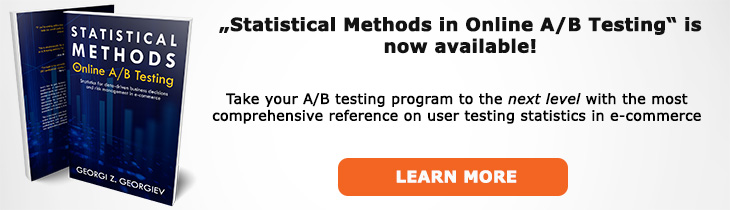# What does "Statistic" mean?

Definition of Statistic in the context of A/B testing (online controlled experiments).

## What is a Statistic?

A statistic is a single measure of an attribute of a sample calculated by applying a function to the set of data that comprises the sample. The function should be independent of the sample's distribution: it should be known beforehand. In estimation a statistic is called an estimator. A statistic should not be mistaken with a parameter since the latter is usually an unknown entity unless the whole population is observable.

The most commonly used statistic is the sample mean, usually the arithmetic mean (geometric or harmonic means can be the appropriate ones in certain cases). As an estimator the sample average is a statistic that estimates the parameter of interest: the population average. For example, the average revenue per user in a sample of n users from a website's traffic in a restricted time period can be used to estimate the actual average revenue per user across a longer time frame under certain assumptions (statistical model).

In A/B testing we perform statistical hypotesis testing with regards to a primary KPI, often a conversion rate of some kind, which is a statistic. In the hypothesis test we use a test statistic such as the z score, t score and the p-value, which is a distance function of the data which measures the discrepancy between a hypothetical and observed value.

Like this glossary entry? For an in-depth and comprehensive reading on A/B testing stats, check out the book "Statistical Methods in Online A/B Testing" by the author of this glossary, Georgi Georgiev.## Glossary Index by Letter

Select a letter to see all A/B testing terms starting with that letter or visit the Glossary homepage to see all.